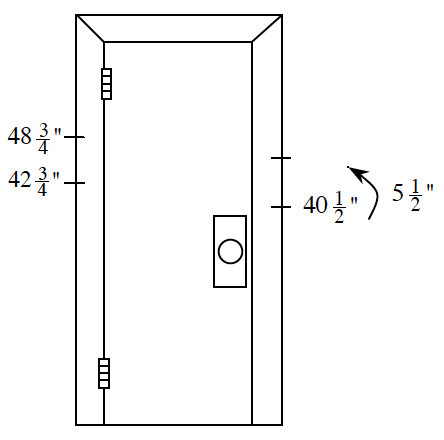### Home > MC1 > Chapter 3 > Lesson 3.1.1 > Problem3-9

3-9.

Kip and Jordan are brothers. Their dad measures them once a year by drawing a line on a doorframe in their house.

1. Kip grew from $42\frac { 3 } { 4 }$ inches to $48\frac { 3 } { 4 }$ inches. How much did he grow last year?

• Subtracting fractions can be tricky, but here we know that

$\frac{3}{4}-\frac{3}{4}=0$

Be sure that you subtract Kip's height this year from Kip's height last year.
If you get this mixed up, you will end up with a negative number!

1. Jordan was $40\frac { 1 } { 2 }$ inches and grew $5\frac { 1 } { 2 }$ inches. How tall is he now?

• Using this information, add the lengths together to find Jordan's height this year.

• Remember, two halves make one whole.

1. Which boy grew more last year?

Compare your answer to part (a) and the information given in part (b).
Can you tell who grew more?

Kip grew more this year.

•